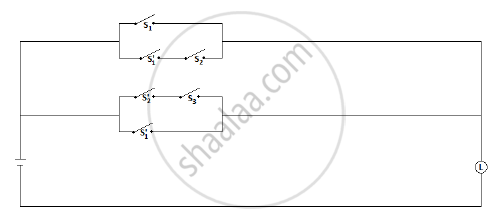HSC Arts 12th Board ExamMaharashtra State Board
Share

# Construct the switching circuit for the following statement : [p v (~ p ∧ q)] v [(- q ∧ r) v ~ p] - HSC Arts 12th Board Exam - Mathematics and Statistics

ConceptMathematical Logic Application - Introduction to Switching Circuits

#### Question

Construct the switching circuit for the following statement : [p v (~ p ∧ q)] v [(- q ∧ r) v ~ p]

#### Solution

Let

• p: the switch S1 is closed.
• q: the switch S2 is closed.
• r: the switch S3 is closed.Is there an error in this question or solution?

#### APPEARS IN

2014-2015 (March) (with solutions)
Question 3.1.3 | 3.00 marks
Solution Construct the switching circuit for the following statement : [p v (~ p ∧ q)] v [(- q ∧ r) v ~ p] Concept: Mathematical Logic - Application - Introduction to Switching Circuits.
S